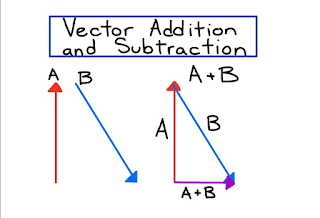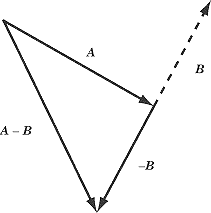Vector Addition and Subtraction - العلم نور

# العلم نور

{ وَقُلْ رَبِّ زِدْنِي عِلْمًا }

## الجمعة، 23 أغسطس 2019Vector Addition and Subtraction

### Vector Definition

Do you see the vectors in the above picture? If yes, what did you observe? There are to vectors A and B. Each vector should have both magnitude and direction to be completely described. Each vector has two main sections; the line and the arrowhead. The magnitude is calculated by knowing the length of this line and the direction of the vector is known by the arrowhead direction.

Vectors addition and subtraction can be done by different methods. In the next section, we will discuss the different methods which can be used to add and subtract vectors in addition to show more answered examples for understanding vector addition and subtraction.

The corresponding components of two vectors have to be added or subtracted to each other in order to add or subtract the two vectors.
Let vector A = (a1, a2), and vector B = (b1, b2),
Then, the sum of the two vectors A & B will be as follows:
A + B = (a1 + b1, a2 + b2)
And, the difference of the two vectors A & B will be as follows:
A – B = (a1 - b1, a2 - b2)
The addition and subtraction of two different vectors can be done using either the parallelogram method or the triangle method. We will discuss the two methods below.

#### parallelogram method

The addition of two different vectors, say A & B can be done by this method as follows:
-        Place the two vectors A and B at the same initial point.
-        Then, complete the parallelogram and the addition result of A & B (A + B) will be the diagonal of this parallelogram.
This method steps are illustrated below:parallelogram method

The subtraction of two different vectors, say U & V can be done by this method as follows:
-        Move the B vector to the opposite direction and change its sign to become -B.
-        Then, complete the parallelogram and the subtraction result of A & B (A - B) will be the diagonal of this parallelogram.

#### Triangle Method

The addition and subtraction of two vectors A & B according to the triangle method will be as follows:
1.    The first step is to place the two vectors one after another, that will be done by placing the initial point of the second vector at the terminal point of the first vector.
2.    The second step is to draw a resultant vector R which connects between the initial point of the first vector and the terminal point of the second vector.
The addition of vectors A & B by this method can be illustrated as follows:Addition by Triangle Method

The subtraction of vectors A & B by this method can be illustrated as follows:Subtraction by Triangle Method

Example
Find A + B, and A – B, if A = (3, 4), B = (5, -1).